International Journal of Data Science and Analysis
Volume 2, Issue 1, October 2016, Pages: 1-6

Extensions of Tests of Indirect Effect in Mediation Analysis

Chike Henry Nwankwo1, Amechi Henry Igweze2

1Department of Statistics, Nnamdi Azikiwe University, Awka, Nigeria

2Department of Mathematics and Statistics, Delta State Polytechnic, Ogwashi-Uku, Nigeria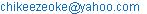(N. C. Henry)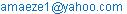(I. A. Henry)

Chike Henry Nwankwo, Amechi Henry Igweze. Extensions of Tests of Indirect Effect in Mediation Analysis. International Journal of Data Science and Analysis. Vol. 2, No. 1, 2016, pp. 1-6. doi: 10.11648/j.ijdsa.20160201.11

Received: July 19, 2016; Accepted: July 27, 2016; Published: September 5, 2016

Abstract: This study seeks to determine economic variables that jointly mediate upon government expenditure and unemployment in Nigeria and in proven cases of mediation, determine the type of mediation that exist. It compares the two methods of calculating indirect effect which are product of coefficient and difference of coefficients respectively. Furthermore, the study compares the three methods of testing the significance of indirect effect vis-à-vis Sobel’s test, Aroian test and Goodman’s test. The differences in these three tests are due to variations in the methods of standard error computation. This study further proposed extensions for the three variations in methods of standard error computation for a case of two mediator which may also be extended for more than two mediator cases. The results show that a combination of any two of labour force population, interest rate and inflation showed a significant evidence of partial mediation. The result of indirect effect computation shows that the method of product of coefficient gave a slightly higher result when compared to that of difference of coefficient. The proposed extension of Sobel, Aroian and Goodman tests gave the same result for all cases as their standard errors are the same. The study thus recommends employing some variable selection techniques in subsequent studies which may improve mediational results; further studies may to be carried out to seek methods of ascertaining the direction of relationship of indirect effect, other than those of the regression models; and finally studies may be carried out to determine the effect of multicolinearity on mediation results.

Keywords: Aroian Test, Sobel Test, Goodman Test, Indirect Effect, Mediation

Contents

1. Introduction

For many decades now, successive governments in Nigeria had adopted various policies to create jobs and reduce unemployment. In spite of these policies, unemployment has continued to rise unabated. This may suggest that there could be other factors mediating between government expenditures and unemployment in Nigeria.

Often times, theory suggests that a third variable may improve understanding of the nature of the relationship between the two primary variables. The third variable considered a mediator, is hypothesized to be linked in a causal chain between the independent and dependent variables. In other words, the independent variable causes the mediator and the mediator causes the dependent variable. The search for intermediate causal variables is called mediation analysis.

Fairchild & MacKinnon (2009) noted that a mediation model is one that seeks to identify and explain the mechanism or process that underlies an observed relationship between an independent variable and a dependent variable via the inclusion of a third explanatory variable, known as a mediator variable.

In mediation analysis, the most common tests of the indirect effect are extensions of the Sobel’s test, which are ratios of the effect size and the standard error of the indirect effect. The indirect effect size in the single-mediator model may be calculated in two ways, as either product of coefficient, âb̂ or difference of coefficient ĉ − ĉ′. The value of the mediated or indirect effect estimated by taking the difference in the coefficients, ĉ − ĉ′, is said to corresponds to the reduction in the independent variable effect on the dependent variable when adjusted for the mediator.

There are three principal versions of the "Sobel test": one that adds the third denominator term,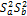; by Aroian, (1947) popularized by Baron & Kenny; one that subtracts the third variable by Goodman, (1960), and one that does not include the third variable at all generally referred to as Sobel test by Sobel (1982). Aroian version of the Sobel test was recommended in Baron and Kenny (1986) because it does not make the unnecessary assumption that the product of Sa and Sb is vanishingly small. The Goodman version of the test subtracts the third term for an unbiased estimate of the variance of the mediated effect, but according to Preacher & Leonardelli (2010), this can sometimes have the unfortunate effect of yielding a negative variance estimate. Again, these Sobel’s test have only been applied to single mediation models. It is still uncertain how the tests will fare when extended to accommodate more than one mediator variables

Purpose of the study

The purpose of the study are:

1.    To obtain the coefficients of double mediation using the product of their unstandardised regression coefficients,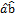and the difference of the regression coefficients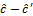and compare the results.

2.    To conduct the Sobel’s test, Aroian test and Goodman test of indirect effect for a double mediator and compare their results

3.    To propose an extension of the Sobel’s test, Aroian test and Goodman test of indirect effect for two mediator models and compare their results

2. Literature Review

Several studies have been carried out on mediation, economic variables, and unemployment and government expenditure. Some of such studies are reviewed.

Tunah (2010) studied the macroeconomic variables which affects unemployment for Turkey. Quarterly data from 2000 to 2008 was used in the study. Augment Dickey Fuller test (ADF), Phillip-Perron test, Johansen’s cointegration, and granger causality techniques were used for analysis. The results of the study showed that there is a significant impact of real GDP, consumer price index and previous unemployment rate on the unemployment rate. Whereas real effective exchange rate has no impact on the unemployment.

Lui (2009) studied the relationship between inflation and unemployment in a situation where inflation has differential outcomes on employed and unemployed. The result shows that the relationship between inflation and unemployment is either negative or positive which depend on goods & labor market institutions. A higher rate of inflation increases workers’ incentives to work & generates a negative effect on unemployment. On the other hand, inflation lowers a firm’s return from creating job vacancies, thereby raising unemployment

Pallis (2006) studied the relationship between inflation and unemployment in new European Union member states. The three variables employed are "the price deflator of gross domestic product at market prices (national currency; annual percentage change)" and "nominal compensation per employee; total economy (national currency; annual percentage change)" and "total employment rate (%)". Non-linear least square regression was employed in the study. The paper concludes that the application of common policies across economy may be questionable because of the different effect of these policies on inflation and unemployment

El-Agrody et al. (2010) examined unemployment and its impact on the GDP in Egypt. Data was collected from the year 1994 to 2004. Linear regression analysis were applied. Variables used in the study were privatization, population, consumption expenditure, interest rates, exchange rates, technology, agricultural domestic product, real wage rates, and agricultural investment. The results showed that there is a significant positive impact of Egypt national unemployment, national investment, exchange rate and average per capita share of GDP on the volume of GDP. The results also highlighted privatization and increasing population as the main reasons of increasing unemployment. They recommended that privatization policies need to be revised and to reduce interest rates in order to lowering the agricultural unemployment.

Doğan (2012) investigated the response of unemployment to selective macroeconomics shocks for the period of 2000:Q1-2010:Q1. It finds that positive shocks to growth, growth in export and inflation reduce unemployment. On the other hand, shocks to exchange rate, interbank interest rate and money supply increase unemployment. The the author states that the results are consistent with Phillips curve and Okun’s Law suggestion. Namely, negative relationship between output and unemployment and positive relationship between unemployment and inflation are found.

The interest of mediation is in calculation the size of the mediating effect also known as indirect effect. The indirect effect measures the extent to which the dependent variable changes when the independent variable is held fixed; and the mediator variable changes by the amount it would have changed had the independent variable increased by one unit (Judd & Kenny, 1981)

The indirect effect measures the extent to which the dependent variable changes when the independent variable is held fixed; and the mediator variable changes by the amount it would have changed had the independent variable increased by one unit (Judd & Kenny, 1981)

According to MacKinnon & Dwyer (1993), the indirect effect in the single-mediator model may be calculated in two ways, as either âb̂ or ĉ − ĉ′. The value of the mediated or indirect effect estimated by taking the difference in the coefficients, ĉ − ĉ′, corresponds to the reduction in the independent variable effect on the dependent variable when adjusted for the mediator. The difference between the coefficients obtained from the two different regression coefficients (ĉ − ĉ′) should be equal to the product of the coefficients ab. To test for significance of indirect effect, the difference is then divided by the standard error of the difference and the ratio is compared to a standard normal distribution.

James et al. (2006) noted that researchers often test whether there is complete or partial mediation by testing whether the c′ coefficient is statistically significant, which is a test of whether the association between the independent and dependent variable is completely accounted for by the mediator. If the c′ coefficient is statistically significant and there is significant mediation, then there is evidence for partial mediation. It is often unrealistic to expect that a single mediator would be explained completely by an independent variable to dependent variable relation.

From the Baron & Kenny (1986) approach, mediation is supported if the partial direct effect for path c is non significantly different from zero and path b is significantly greater than zero.. If c is non significantly different from zero, results are consistent with a full mediational model. If path b is significant after controlling for the direct effect of X (path c), but path c is still significant, the model is consistent with partial mediation.

Many studies investigating mediation according to MacKinnon, Fairchild & Fritz (2007) use a randomized experimental design, where participants are randomized to levels of one or more factors in order to demonstrate a pattern of results consistent with one theory and inconsistent with another theory Differences in means between groups are then attributed to the experimental manipulation of the mediator. The results of the randomized study along with the predictions of different theories are used to provide evidence for a mediation hypothesis and suggest further studies to localize and validate the mediating process.

Milovanović (2013) showed the procedure for researching hidden influence of predictor variables in regression models and depicting suppressor and mediator variables. The author also showed that detection of suppressor and mediator variables could provide refined information about the research problem.

Suradi & Ali et al (2009) investigated how the third variable can be determined whether it functions as a full mediator or a partial mediator in a relationship analysis between independent variable and dependent variable. A mediator serves as a third variable that alters the relationship between an independent variable and a dependent variable. Inclusion of a mediator in research allows researchers to more-precisely consider the explanations of the relationship between independent and dependent variables.

Imai et al (2010) further affirmed that statistical independence of potential outcomes and potential mediator is assumed. When multiple regression models are used, the mediators are assumed independent.

Preacher & Hayes (2008) however argued that interpretation of the mediation analysis does not focus at all on the statistical significance of the a and b paths, as is required using the causal steps method. Instead, emphasis is placed almost entirely on the size and significance of the indirect effect.

Oyeka & Nwankwo (2014) proposed and developed the use of the non-cummulative dummy variables of 1’s and 0’s to represent levels of parent independent variables in dummy variable multiple regression models. The regression coefficients obtained using their proposed methods were easier to interpret and clearly understood than the use of the cumulatively coded ordinal dummy variables of 1’s and 0’s that could be used for the same purpose. Their method also enables the simultaneous estimation of the total, absolute or overall effect of a parent independent variable as well as its direct effect through its representative dummies and its indirect effect on a given independent variable through the mediation of other parent independent variables in the model was demonstrated. They found that the indirect effect of a given parent independent variable on a dependent variable is the difference between its total or absolute effect and its direct effect through its representative dummy variables. The total or absolute effect itself is the simple regression coefficient or regression effect of the parent independent variable using directly its assigned numerical codes on the dependent variable. Thus the indirect effect of the parent independent variable X, on a dependent variable Y through the mediation of other parent independent variables in the model is estimated as its total or absolute effect less its direct effect

Sherman & Gorkin (1980) randomly assigned subjects to solve either (a) a sex- role related brainteaser, or (b) a brainteaser not related to sex roles. The sexist brainteaser condition was designed to evoke cognitive dissonance in the self-identified feminist subjects, while the nonsex-role related condition was not. Participants were then asked to judge the fairness of a legal decision made in an affirmative action trial. The results were consistent with the prediction that participants with strong feminist beliefs were more likely to make extreme feminist judgments in the trial if they failed the sexist brainteaser task, in an attempt to reduce cognitive dissonance. Although results of this experiment were taken as evidence of a cognitive dissonance mediation relation, the mediating variable of cognitive conflict was not measured to obtain more information on the link between the manipulation, cognitive dissonance, and feminist judgments.

According to Small (2013), researchers are often interested in mediation analysis to understand how a treatment works, in particular how much of a treatment’s effect is mediated by an intermediated variable and how much the treatment directly affects the outcome not through the mediator. The standard regression approach to mediation analysis assumes sequential ignorability of the mediator, which is that the mediator is effectively randomly assigned given baseline covariates and the randomized treatment. The author argued that since the experiment does not randomize the mediator, sequential ignorability is often not plausible.

MacKinnon, Lockwood, and Williams (2004) also compared the bootstrap resampling method with the single sample method and found that the bootstrap method obtained more accurate confidence limits. They further suggested that confidence limits of the mediation effects provided much more information than the estimates themselves.

3. Research Methods

The study employs mediation analysis. The analysis begins by testing for multicolinearity among the independent variables. This was accomplished with the use of variance Inflation Factor (VIFk). The first step in meditational analysis is to establish that a relationship exist between the independent variable and the dependent variable using a linear regression model. This is known as path c. Next is to conduct a regression analysis with the suspected variables predicting the dependent variable. This is known as path b. thirdly, conduct a regression analysis with the independent and other variables predicting the dependent variable, known as partial effect model.

MacKinnon (2000) listed three major approaches to statistical mediation analysis: (a) causal steps (b) difference in coefficients, and (c) product of coefficients All of these methods use information from the three of the following regression equations:

Y=c0+cX                                      (1)

E (Z) = a0+aX                                 (2)

E (Y)= c0 + cX+ bZ                      (3a)

E (Yi)= c0ij+ cijX+ biZi+ bjZj; i < j ≤ m              (3b)

where c0, a0, c0i and c0ij are intercepts of (1), (2), (3a) and (3b)

Y is the dependent variable; X is the independent variable; Zi is the ith mediator,

c0, c0i and c0ij are the coefficients relating the independent variable and the dependent variable in (1), (3a) and (3b) respectively.

ai is the coefficient relating the independent variable and ith mediator variable in (2).

bi and bj are the coefficients relating the ith and jth mediators respectively to the dependent variable in the partial effect models in (3a) and (3b).

To determine the type of mediation, Baron & Kenny (1986) stated that if c is non significantly different from zero while bi is significantly greater than zero, results are consistent with a full mediation. If bi is significant after controlling for the direct effect of X, but path ci is still significant, the model is consistent with partial mediation. The aim of mediation analysis is to calculate and test for the significance of mediational effect known as indirect effect.

The test of significance of Total effect

The procedure below test the significance of the regression parameters in the regression models.

Test hypothesis is H0: C=0 vs H1: C≠0

The test statistics is given as: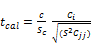(4)

at α=0.05 and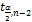Where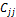is the diagonal element of (X′X)-1 and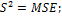Judd & Kenny (1981) outlined a procedure for calculating indirect effect. This is obtained by subtracting coefficient of X in equation (3a) or (3b) (depending on the number of mediators) from the coefficient of X in equation (1):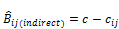(5)

Proposed Test of Significance of the Indirect Effect for two mediators

The proposed test of significance of the indirect effect for the two mediator model can be constructed using a ratio of the indirect effects to their standard errors as in the single mediator cases. The extension is predicated on the statistical fact that the variance of a sum of two random variables is equal to the sum of their individual variances when the variables are independent.

It is worthy of note here that pooling the standard error of the path coefficients is not needful as it is already pooled by the inclusion of the sample variances, Sai2and Sbi2in the single cases respectively (Preacher & Kelly, 2011)

Hypothesis:

H0: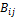= 0 Versus H1:≠ 0

Extended Sobel’s test:

The extended Sobel’s test of indirect effect for two mediators (i, j) is given as: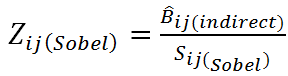(6a)

Where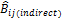is the indirect effect of the ith and jth mediator in (5).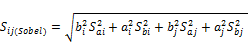(6b)

Extended Aroian test:

The extended Aroian test of indirect effect for the ith and jth mediators is given as: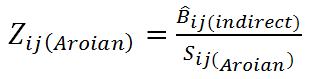(7a)

Where: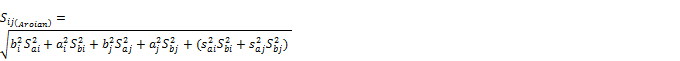(7b)

Extended Goodman Test:

The extended Goodman’s test of indirect effect for the ith and jth mediators is given as:(8a)

Where:(8b)

bi and bj are the unstandardized coefficient of Zi and Zj in the partial effect model in equation (3b).

ai and aj are the unstandardized coefficient of X when when testing for the significance of two mediators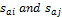are the standard errors of ai and aj respectively while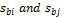are the standard error of bi and bj respectively.

Decision Rule:

If p-value is less than α = 0.05, reject H0 and conclude the of significant indirect effect.

4. Results and Discussion of Findings

The single mediator analysis is the basis on which the two mediator case has been conducted. Here single mediators that were originally significant and satisfied the assumptions of single mediation are paired without replacement and subsequently used in the two mediator cases.

Partial effect model for two mediator Variable

The condition for existence of mediation in the two mediator cases is that the coefficient of X in the partial effect model, cij must be less than the coefficient of X in path c. In this regards we have only two mediators, labour force population (Z3) and inflation (Z6).

Thus, the partial effect model is the model of both independent variable and the two mediator variables Z3 and Z6 predicting the dependent variable.

The various coefficients in the partial effect model for the two mediator case are present in table 4.1 below:

Table 1. Coefficients and standard errors of partial effect model.

 Zi, Zj bi bj cij Sbi Sbj Scij P(Cij) p(bi) p(bj) Z3, Z6 1.497 -0.06 5.53×10-13 0.733 0.029 0 0 0.018 0.043

Indirect effect for two mediator cases

The indirect effect when two mediator are used are presented in table 4.2 below.

Table 2. Indirect effect sizes.

 Zi, Zj c bi bj cij c-cij ai aj aibi ajbj aibi+ajbi Z3, Z6 6.13x10-13 1.497 -0.06 5.53 x10-13 6.03 x10-14 1.87x10-14 -5.50x10-13 2.79 x10-14 3.29 x10-14 6.08 x10-14

From the results presented above, it is seen that the method of product of coefficients (aibi+ajbj) gave a slightly higher effect size when compared to the method of difference of coefficient (c-cij)

Extended Standard error of effect size.

Sobel’s Test:

The Extended Sobels standard error of effect size is calculated from equation (14b) as follows: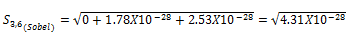= 2.08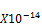The Sobels standard error of effect size computation for two mediator cases is summarized in table below:

Table 3. Extended Sobels Standard error.

 Zi, Zj Sai Saj Sbi Sbj bi2Sai2 ai2Sbi2 bi2Saj2 aj2Sbj2 Sij(Sobel) Z3, Z6 0 0 0.733 0.029 0 1.87x10-28 0 2.53x10-28 2.08 x10-14

since Sai = 0 then Sai2Sbi2 = 0, similarly since all Saj = 0 then Saj2Sbj2 = 0 it implies that extended standard error of effect size for Aroian and Goodman will be the same as Sobel test.

Test of significance of indirect effect

The indirect effect for two mediator is tested with the aid of equation (8a)

Extended Sobels test:

Table 4.4 gives the result of the Extended Sobels test in equation (8a). The summary of the test of significance of the indirect effect is presented in table 4.4

Table 4. Test of significance of indirect effect (c-cij).

 Zi, Zj Bij(Sobel) Sij(Sobel) Zij(Sobel) P-value Z3, Z6 6.03 x10-14 2.08 x10-14 2.899 0.004

Table 5. Test of significance of indirect effect (aibi+ajbi).

 Zi, Zj aibi+ajbi Sij(Sobel) Zij(Sobel) P-value Z3, Z6 6.08 x10-14 2.08 x10-14 2.923 0.0037

From the result presented in table 4.4 and 4.5, the method of product of coefficient was seen to have the greater Effect size and smaller p-value than the method of difference of coefficient. This variation also may be due to rounding. The pair of mediator variables Z3 and Z6 was found to jointly mediate between unemployment rate and government expenditure.

5. Conclusion

This study compares various methods of test of indirect effect in mediations analysis. Firstly, it compares the two methods of calculating indirect effect which are product of coefficients and difference of coefficients. This study also proposes extentions for the three tests of mediational effect for a case of two mediator which may also be extended for more than two mediator cases. The study further seeks to determine economic variables that mediate upon government expenditure and unemployment in Nigeria for double mediation, and in proven cases of mediation, determine the type of mediation that exist. The following were the findings made in this study:

The comparison of two methods of calculating indirect effect show that both methods of product of coefficients and difference of coefficients give approximately the same result. However the product of coefficient gave a slightly higher result, which may be as a result of rounding errors;

The proposed extension of Sobel, Aroian and Goodman tests gave the same result as their standard errors are the same; In determine economic variables that mediate upon government expenditure and unemployment in Nigeria, labour force population (Z3) and inflation (Z6) were found to partially mediate upon government expenditure and unemployment in Nigeria: Although not statistically significant, labour force population (Z3) and inflation (Z6) were respectively found to have trace of partial mediation in the single mediator case. In economic views, increase in labour force population without proportionate increase in job creation directly increases unemployment rate in a country. Again high rate of inflation, which is persistent increase in the prices of goods and services creates additional costs of labour and thus indirectly reducing the number of man power that can be demanded for. Also high cost of goods and services increase the cost of starting up as well as sustaining existing businesses and thus reducing the number of man power than can be employed.

This study presents a clear case where the product of Sa and Sb is equal to Zero. Hence the Sobels test is not only recommended when the products of Sa and Sb is vanishingly small, but also when they are zero.

References

1. Asif, K (2013). Factors Effecting Unemployment: A Cross Country Analysis. International Journal of Academic Research in Business and Social Sciences, Vol. 3(1).
2. Congress Of The United States Congressional Budget Office, CBO (2012) Understanding and Responding to Persistently High Unemployment.
3. Doğan, T. T (2012). Macroeconomic Variables and Unemployment: The Case of Turkey. International Journal of Economics and Financial Issues Vol. 2(1).
4. El-Agrody, N. M., Othman, A. Z., & Hassan, M. B.-D. (2010). Economic Study of Unemployment in Egypt and Impacts on GDP. Nature and Science, 8 (10), 102-111.
5. Imai, K.; Keele, L. & Tingley, D. (2010). A General Approach to Causal Mediation Analysis.' Psychological Methods, Vol. 15, No. 4 (December), pp. 309-334. International statistics p.
6. Judd CM, Kenny DA. (1981)Process analysis: Estimating mediation in treatment evaluations. Evaluation. Review. (5) pp: 602–619.
7. Lui, L. Q. (2009). Inflation and Unemployment: The roles of goos and labor market institution. 1 31.
8. Milovanović, B (2013) About Hidden Influence Of Predictor Variables-Suppressor And Mediator Variables. J. Geogr. Inst. Cvijic. 63(2) (1-10).
9. Oyeka, I. C. A., Nwankwo, C H (2014). Direct and Indirect Effects in Dummy Variable Regression. American Journal of Theoretical and Applied Statistics. Vol. 3, No. 2, 2014, pp. 44-48. doi: 10.11648/j.ajtas.20140302.
10. Pallis, D. (2006). The tradeoff between inflation and unemployment in new European Union member-states. International Research Journal of Finance and Economics (1), 81-97.
11. Preacher, K. J., & Hayes, A. F. (2008). Contemporary approaches to assessing mediation in communication research. In A. F. Hayes, M. D.
12. Sobel, M. E. (1982). Asymptotic Confidence Intervals for Indirect Effects in Structural equations models. In S. Leinhart (Ed.), Sociological methodology 1982 (pp. 290-312). San Francisco: Jossey-Bass.
13. Sobel, M. E. (1986). Some new results on indirect effects and their standard errors in covariance structure models. In N. Tuma (Ed.), Sociological Methodology 1986 (pp. 159-186). Washington, DC: American Sociological Association.
14. Suradi, N. R. M, Ali, Z. M; Alwi, H. Z. M; Norafidah, S and Ramli, M (2009) Testing For Mediating Variable In A Regression Model. International Conference on Quality, Productivity and Performance Measurement.
15. Tunah, H. (2010). The Analysis of Unemployment in Turkey: Some Empirical Evidence Using Co integration Test. European Journal of Social Sciences, 18 (1), 18-38.

 Contents 1. 2. 3. 4. 5.
Article ToolsAbstractPDF(222K)